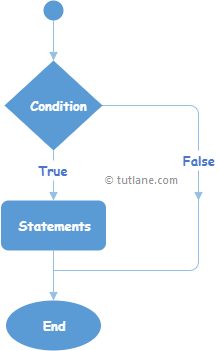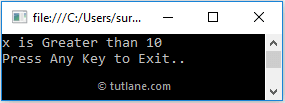# Visual Basic If Statement

In Visual Basic, If statement is useful to execute the block of code or statements conditionally based on the value of an expression.

Generally, in Visual Basic, the statement that needs to be executed based on the condition is known as a “Conditional Statement” and the statement is more likely a block of code.

## Syntax of Visual Basic if Statement

Following is the syntax of defining the if statement in Visual Basic programming language.

If bool_expression Then
// Statements to Execute if condition is true
End If

If you observe the above If statement syntax, the statements inside of the If condition will be executed only when the “bool_expression” returns true otherwise, those statements will be ignored for execution.

Following is the sample example of using the If statement in Visual Basic programming language.

Dim x As Integer = 20
If x >= 10 Then
Console.WriteLine("Number Greater than 10")
End If

If you observe the above example, the Console statement will be executed only when the defined condition (x >= 10) returns true.

## Visual Basic If Statement Flow Chart Diagram

Following is the flow chart diagram, which will represent the process flow of the If statement in Visual Basic programming language.If you observe the above Visual Basic If statement flow chart diagram, when the defined condition is true, the statements within the If condition will be executed; otherwise, the If condition will end without executing the statements.

## Visual Basic If Statement Example

Following is the example of defining the If statement in Visual Basic programming language to execute the block of code or statements based on a Boolean expression.

Module Module1
Sub Main()
Dim x As Integer = 20, y As Integer = 10
If x >= 10 Then
Console.WriteLine("x is Greater than 10")
End If
If y <= 5 Then
Console.WriteLine("y is less than or equals to 5")
End If
Console.WriteLine("Press Enter Key to Exit..")
End Sub
End Module

If you observe the above example, we defined two If conditions to execute the statements based on the defined variables (x, y) values.

When we execute the above Visual Basic program, we will get the result as shown below.If you observe the above result, only one If condition is true that’s the reason only one statement printed on the console window.

This is how we can use the If statement in Visual Basic programming language to execute the block of code or statements based on our requirements.